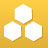cancel
Showing results for
Search instead for
Did you mean:New Contributor

## Le Chatelier's Principle

How does Le Chatelier's Principle deal with a change in reaction conditions which can simultaneously effect the equilibrium in opposite ways.

For example, here's the equation for the conversion of N2 and H2 into NH3. It's an exothermic reaction, so I've added heat as a product on the right side.

N2(g) + 3H2(g) <-> 2NH3(g) + heat

If I increase the temperature (add heat) that would tend to push the reaction to the left because the reaction to the right is an exothermic reaction. But if I add heat (and the volume remains constant), then I'm also increasing the pressure, which should tend to push the reaction to the right, towards fewer moles of gaseous products.

Is there a simple explanation for how Le Chatelier's Principle works in such a situation?

Thanks.

Tags (2)